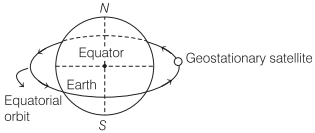# Earth’s Satellite | Definition, Formulas, Types – Gravitation

Satellite communication:
A heavenly object which revolves around a planet is called a satellite.

We are giving a detailed and clear sheet on all Physics Notes that are very useful to understand the Basic Physics Concepts.

## Earth’s Satellite | Definition, Formulas, Types – Gravitation

Natural satellites:
Natural satellites are those heavenly objects which are not man made and revolves around the earth. Artificial satellites are those heavenly objects which are man made and launched for some purposes and revolve around the earth.

Time period of satellite, T = $$\frac{2 \pi r}{\sqrt{\frac{G M}{r}}}$$

Here, r = radius of orbital of satellite.

After simplifying, T = $$2 \pi \sqrt{\frac{r^{3}}{G M}}=\frac{2 \pi}{R} \sqrt{\frac{(R+h)^{3}}{g}}$$

[∵ g = $$\frac{G M}{R^{2}}$$]

where, R = radius of earth,
and h = height of satellite above surface of earth.
Near the earth surface, time period of the satellite

$$T=2 \pi \sqrt{\frac{R^{3}}{G M}}=\sqrt{\frac{3 \pi}{G \rho}}$$
$$T=2 \pi \sqrt{\frac{R}{g}}$$ = 5.08 x 103s = 84 min ≈ 1.4 h

where, Ρ is the average density of earth.
Artificial satellites are of two types

Geostationary Satellite Communication or Parking Satellites:
A satellite which appears to be at a fixed position at a definite height to an observer on earth is called geostationary or parking satellite.Height, from earth’s surface = 36000 km
Radius of orbit = 42400 km
Time period = 24 h
Orbital velocity = 3.1 km/s
Angular velocity = $$\frac{2 \pi}{24}=\frac{\pi}{12}$$ rad/h

• These satellites revolve around the earth in equatorial orbits.
• The angular velocity of the satellite is same in magnitude and direction as that of angular velocity of the earth about its own axis.
• These satellites are used in communication purpose.
• INSAT 2B and INSAT 2C are geostationary satellites of India.

Polar Satellites:
These are those satellites which revolve in polar orbits around earth. A polar orbit is that orbit whose angle of inclination with equatorial plane of earth is 90°.Polar Satellite Height from earth’s surface ≈ 880 km
Time period ≈ 84 min
Orbital velocity = 8 km/s
Angular velocity = $$\frac{2 \pi}{84}=\frac{\pi}{42}$$ rad/min

• These satellites revolve around the earth in polar orbits.
• These satellites are used in forecasting weather, studying the upper region of the atmosphere, in mapping etc.
• PSLV series satellites are polar satellites of India.

Gravitation:
Have you ever thought, when we throw a ball above the ground level, why it returns back to the ground. It’s because of gravity. When a ball is thrown above the ground in the opposite direction, a gravitational force acts on it which pulls it downwards and makes it fall. This phenomena is called gravitation.

Learn relation between gravitational field and potential field, Kepler’s law of planetary, weightlessness of objects in absence of gravitation, etc.